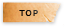# 工程數學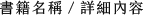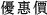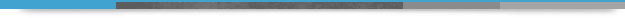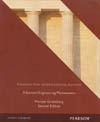## Advanced Engineering Mathematics 2/e (PNIE)

+作者：

### Greenberg

+年份：
2014 年2 版
+ISBN：
9781292042541
+書號：
MA0376P
+規格：

+頁數：
1344
+出版商：
Pearson(Asia)

\$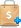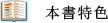• Develops a deep understanding of essential principles as well as hands-on/how-to knowledge of actual practices.
• Serves as an excellent reference tool for both students and practitioners with coverage reaching well beyond Ordinary Differential Equations.
• Reflects the author's vast engineering background, making mathematical principles and applications more relevant to future engineers. Physical applications integrated throughout the text include...
• Harmonic oscillator systems.

• Discussion of beats.

• Application of rank to stoichiometry and dimensional analysis.

• Designs pedagogy with the needs of both instructors and students in mind, combining technical rigor with clarity and accessibility.
• Features a wealth of diverse exercise and problem sets to both challenge and reinforce students' understanding.
• Provides unique Closure features at the end of each section and chapter reviews at the end of each chapter to review and summarize main points.
• Includes useful and unique topics often ignored by other texts, including...
• Dimensional analysis to minimize parameters and guide plots and experiments.

• Introduction to singular integrals.

• Application of the mathematical concept of nonlinearity to nerve impulse and visual perception.

• An “omega method” for the derivation of the various space derivatives of base vectors in non-Cartesian coordinate systems.

• Updates and improves coverage throughout the text to reflect the latest trends in the field and make the learning process more effective and efficient.
• Opens coverage with seven chapters on Ordinary Differential Equations offering flexibility for use in either ODE dedicated courses or combined ODE/Linear Algebra courses.
• Incorporates the Maple Computer Algebra System in the form of optional special-interest sections and exercises.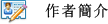Michael GreenbergI. ORDINARY DIFFERENTIAL EQUATIONS.
1. Introduction to Differential Equations.
2. Equations of First Order.
3. Linear Differential Equations of Second Order and Higher.
4. Power Series Solutions.
5. Laplace Transform.
6. Quantitative Methods: Numerical Solution of Differential Equations.
7. Qualitative Methods: Phase Plane and Nonlinear Differential Equations.
II. LINEAR ALGEBRA.
8. Systems of Linear Algebraic Equations; Gauss Elimination.
9. Vector Space.
10. Matrices and Linear Equations.
11. The Eigenvalue Problem.
12. Extension to Complex Case (Optional).
III. SCALAR and VECTOR FIELD THEORY.
13. Differential Calculus of Functions of Several Variables.
14. Vectors in 3-Space.
15.Curves, Surfaces, and Volumes.
16. Scalar and Vector Field Theory.
IV. FOURIER SERIES AND PARTIAL DIFFERENTIAL EQUATIONS.
17. Fourier Series, Fourier Integral, Fourier Transform.
18. Diffusion Equation.
19. Wave Equation.
20. Laplace Equation.
V. COMPLEX VARIABLE THEORY.
21. Functions of a Complex Variable.
22. Conformal Mapping.
23. The Complex Integral Calculus.
24. Taylor Series, Laurent Series, and the Residue Theorem.
Appendix A: Review of Partial Fraction Expansions.
Appendix B: Existence and Uniqueness of Solutions of Systems of Linear Algebraic Equations.
Appendix C: Table of Laplace Transforms.
Appendix D: Table of Fourier Transforms.
Appendix E: Table of Fourier Cosine and Sine Transforms.
Appendix F: Table of Conformal Maps.